# Tensor product of connections

## Definition

Suppose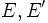$E,E'$ are vector bundles over a differential manifold$M$. Suppose$\nabla$ is a connection on$E$ and$\nabla'$ is a connection on$E'$. The tensor product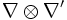$\nabla \otimes \nabla'$ is defined as the unique connection on$E \otimes E'$ such that the following is satisfied for all sections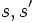$s,s'$ of$E,E'$ respectively: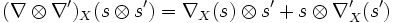$(\nabla \otimes \nabla')_X(s \otimes s') = \nabla_X(s) \otimes s' + s \otimes \nabla'_X(s')$.

## Properties

### Well-definedness

Further information: Tensor product of connections is well-defined

It is not completely clear from the definition that the tensor product of connections is well-defined. What needs to be shown is that the definition given above for pure tensor products of sections can be extended to all sections consistently, while maintaining the property of being a connection.

### Associativity

Further information: Tensor product of connections is associative upto natural isomorphism

Suppose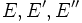$E,E',E''$ are vector bundles over a differential manifold$M$, with connections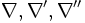$\nabla,\nabla',\nabla''$ respectively. Then, under the natural isomorphism: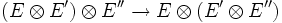$(E \otimes E') \otimes E'' \to E \otimes (E' \otimes E'')$,

the connections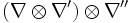$(\nabla \otimes \nabla') \otimes \nabla''$ and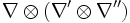$\nabla \otimes (\nabla' \otimes \nabla'')$ get identified.

### Commutativity

Further information: Tensor product of connections is commutative upto natural isomorphism

Suppose$E,E'$ are vector bundles over a differential manifold$M$, with connections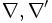$\nabla,\nabla'$ respectively. Then, under the natural isomorphism: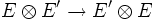$E \otimes E' \to E' \otimes E$

the connections$\nabla \otimes \nabla'$ and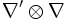$\nabla' \otimes \nabla$ get identified.

### Distributivity with direct sum

Further information: Distributivity relation between direct sum and tensor product of connections

Suppose$E,E',E''$ are vector bundles over a differential manifold$M$, with connections$\nabla,\nabla',\nabla''$ respectively. Then, under the natural isomorphism:$E \otimes (E' \oplus E'') \to (E \otimes E') \oplus (E \otimes E'')$

we have an identification between$\nabla \otimes (\nabla' \oplus \nabla'')$ and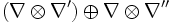$(\nabla \otimes \nabla') \oplus \nabla \otimes \nabla''$. Here,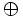$\oplus$ is the direct sum of connections.

An analogous distributivity law identifies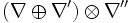$(\nabla \oplus \nabla') \otimes \nabla''$ and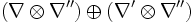$(\nabla \otimes \nabla'') \oplus (\nabla' \otimes \nabla'')$.

### Commutes with dual connection operation

Further information: Dual connection to tensor product equals tensor product of dual connections

## Relation with other interpretations of connection

### Tensor product of module structures

A connection on a vector bundle can be thought of as an interpretation of its global sections as a module over the connection algebra. With this interpretation, a tensor product of connections corresponds to the tensor product of these modules over the connection algebra.

### Tensor product of connections viewed as splittings

Fill this in later

## Facts

### Formula for Riemann curvature tensor

Further information: Formula for curvature of tensor product of connections